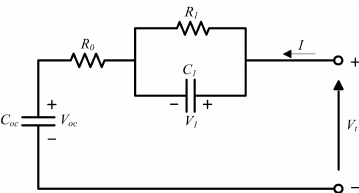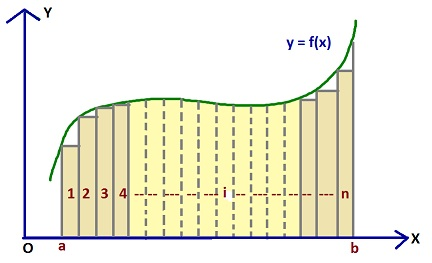# How to Create a Battery Simulator

Standard

Batteries are everywhere. Monitoring and controlling of batteries require smart battery management systems (BMS). To test BMS, one can hook up a real battery, or a simulated one.  Battery simulator is generally more advantageous because it can run faster than realtime, and can induce faults not easily achievable using real batteries. In this article, I will introduce the four steps to create a battery simulator.

## Step 1: Define the battery model

At the core of a simulator is the battery model–a mathematical representation of the battery’s electro-chemical process.  The math model is defined by a set of states, interconnects and parameters.  There are two types of models: steady-state and dynamic.  Steady-state models do not model a battery’s transient behavior and therefore have limited use.  Dynamic models can model the transient behavior if it has sufficient fidelity.  In this article, I will focus on the dynamic models.A common approach to dynamic modeling is to use an equivalent circuit. If the circuit parameters can be determined, well-known circuit analysis can be applied to determine the input-output relationship. Other approaches include modeling the dynamics of battery’s ionic exchange, and applying system identification using neural networks and fuzzy logic.   Since battery is a part of the electrical system, it is more intuitive to model the battery as an equivalent circuit.

## Step 2: Implement a numerical integrator

Dynamic models are described as differential equations of the state variables, such as voltage, current and state-of-charge (SOC). To determine the state variables online, the differential equations need to be integrated. There are a number of numerical integrators, ranging from simple trapezoidal integration to adaptive step-size Runga-Kutta.  The integration function needs to be called repeatedly during simulation, therefore it is usually placed within a program loop or a timer interrupt handler.Numerical integration can become unstable if the step size is too large.  Smaller step size means the simulation may run too slowly. As a general rule, one should start with a small step size to ensure that the model is implemented correctly, then gradually increase the step size without introducing instability.

## Step 3: Extract model parameters from actual data

A battery model is only as accurate as its parameters. The parameters determine both the transient and steady state response.  If a specific battery chemistry is to be modeled, several batteries of the same chemistry should be characterized to extract the model parameters.  The parameter extraction process involves collecting the battery’s current, voltage and temperature data as the battery charges and discharges. Then the data is fitted to the math model using the least square method or something equivalent, or by solving the equivalent circuit equations.

## Step 4: Verify the simulator against real batteries

With the model parameters extracted, the model is ready to be tested.  To verify the model against real batteries, one should first feed the model input data used in the parameter extraction process and see if the model produces similar results as the actual battery output. If that is verified, then test the model against the same battery for other operating conditions.

## Final Thoughts

A battery simulator can be a powerful tool for developing new algorithms and testing the effectiveness of battery management systems under various operating and fault conditions.   However, one must be aware of the simulator’s limitation, and frequently verify the simulation results against the real data.